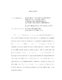## Progress Toward Classifying Teichmueller Disks with Completely Degenerate Kontsevich-Zorich Spectrum2012
##### Authors
Aulicino, David
We present results toward resolving a question posed by Eskin-Kontsevich-Zorich and Forni-Matheus-Zorich. They asked for a classification of all $\text{SL}_2(\mathbb{R})$-invariant ergodic probability measures with completely degenerate Kontsevich - Zorich spectrum. Let $\mathcal{D}_g(1)$ be the subset of the moduli space of Abelian differentials $mathcal{M}_g$ whose elements have period matrix derivative of rank one. There is an $\text{SL}_2(\mathbb{R})$-invariant ergodic probability measure $nu$ with completely degenerate Kontsevich-Zorich spectrum, i.e. $lambda_1 = 1 > lambda_2 = cdots = lambda_g = 0$, if and only if $nu$ has support contained in $\mathcal{D}_g(1)$. We approach this problem by studying Teichm"uller disks contained in $\mathcal{D}_g(1)$. We show that if $(X,omega)$ generates a Teichm"uller disk in $\mathcal{D}_g(1)$, then $(X,omega)$ is completely periodic. Furthermore, we show that there are no Teichm"uller disks in $\mathcal{D}_g(1)$, for $g = 2$, and the known example of a Teichm"uller disk in $mathcal{D}_3(1)$ is the only one. Finally, we present an idea that might be able to fully resolve the problem.